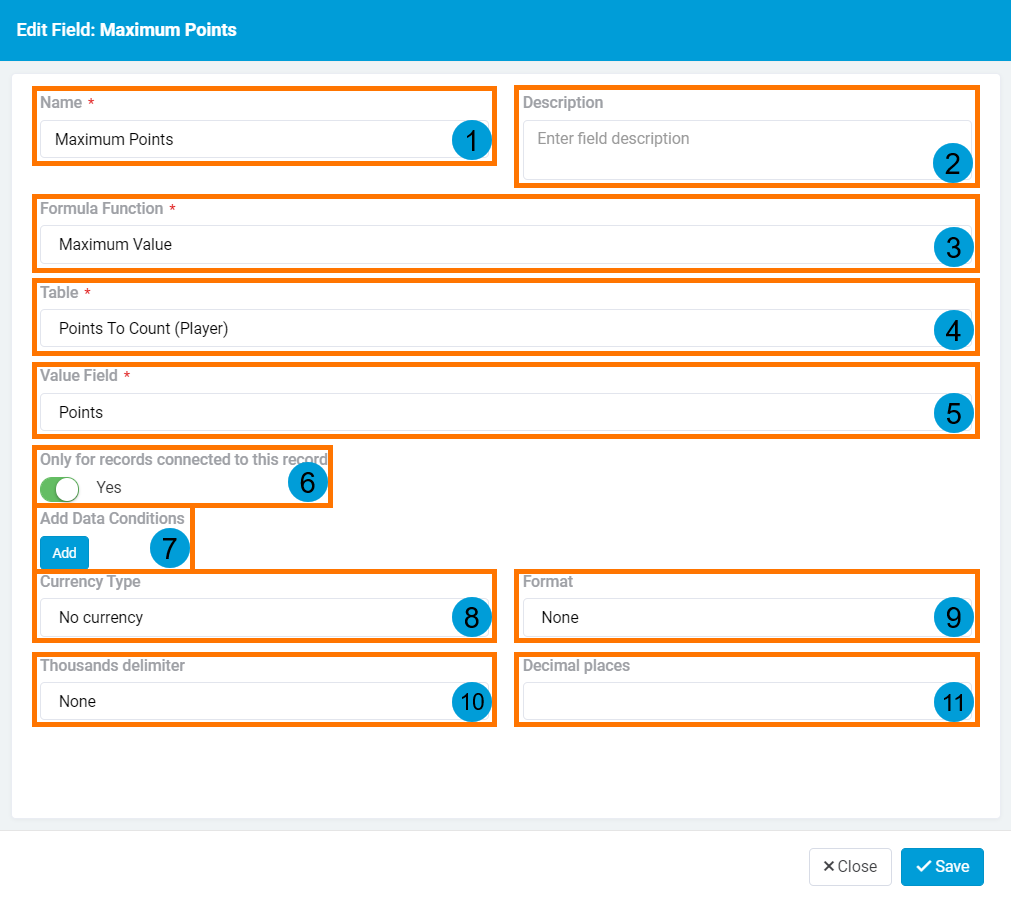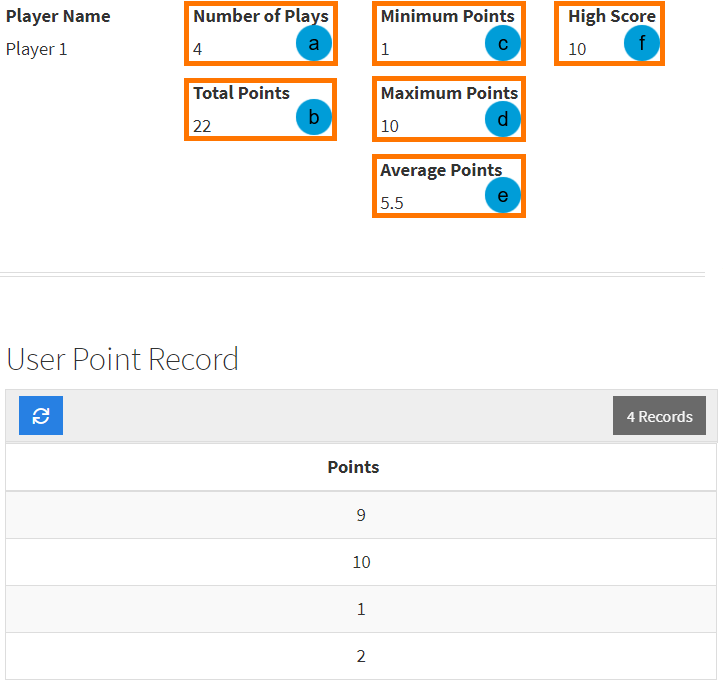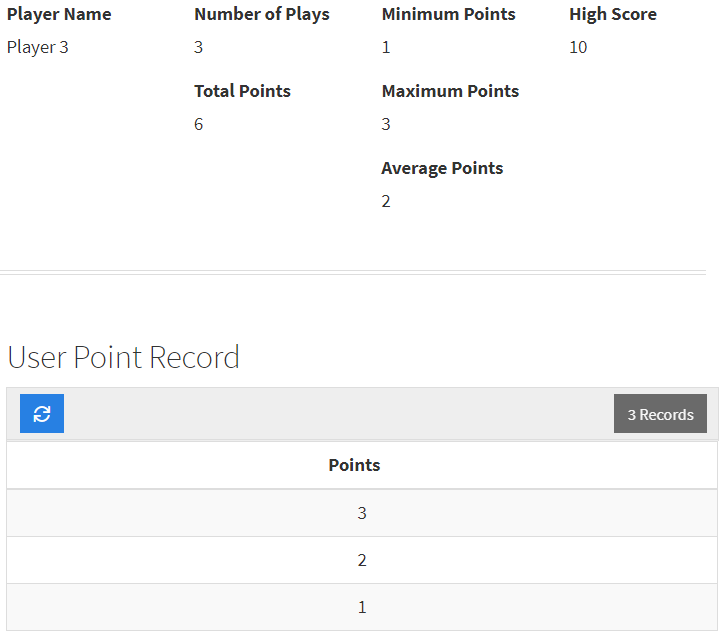# We have moved our support docs to a new location.

Count Fields, Maximum Fields, Minimum Fields, Average Fields, and Sum Fields are subsets of the Complex Formula Field. This type of field is designed to store values to summarize a specified portion of your data.1. The Name for your currency field will help define what this value represents.
2. Descriptions can be a good tool to keep track of further information to describe the field.
3. The Formula Function defines the operation that is done using this field. These options correspond to the value that is to be generated. Available functions are as follows: Count Records, Minimum Value, Maximum Value, Average Value, Sum Value.
4. The Table selected will be the table where the data used in the Formula Function is to be referenced from. This table must be connected to the table which the Complex Formula Field exists in. Read ahead for more information on this portion of the field.
5. The Value Field is the connected field where the function in your field is to be performed on. This portion of the field is not used for the Count Records function.
6. When Only for records connected to this record is set to Yesthe generated value will include only records connected to the given record where setting this option to No includes all records in the connected table. Read ahead to see examples displaying how you can use this setting.
7. Adding Data Conditions to your field can filter the records included in your function based on the values of fields in the connected table.
8. The Currency Type can be selected based on the type of currency that you need to store.
9. The Format determines the location of the currency symbol in components.
10. The Thousands Delimiter can be configured to show the specified symbol to separate groups of thousands.
11. Decimal Place values can be configured to show the specified number of digits after the decimal in your fields.

To display the different types of fields that you can create using the Complex Formula Field, let's take a look at a simple example. This example is a simple score tracker for players of a game.

Let's say we have some players and we would like to summarize their play details. We are given records connected to each player representing the number of points earned for each play.1. Number of Plays is a field generated using the Count Records function. This function counts the number of records connected. In this case, the player has 4 point records.
2. Total Points is a field generated using the Sum Value function. This function counts the total of the value within the connected records. In this case, the player's points across 4 records total to 22.
3. Minimum Points is a field generated using the Minimum Value function. This function finds the smallest value from the connected records. In this case, the player scored 1 point as their lowest score.
4. Maximum Points is a field generated using the Maximum Value function. This function finds the largest value from the connected records. In this case, the player scored 10 points as their highest score.
5. Average Points is a field generated using the Average Value function. This function calculates the average of the connected records.
6. High Score is a field generated from the Maximum Value function. The difference between this field and Maximum Points is that this field has Only for records connected to this record set to No. This setting means that this field finds the highest point value out of all players.

An additional example of player details can be viewed below. Here, we can observe the above functions performed on a different set of data.